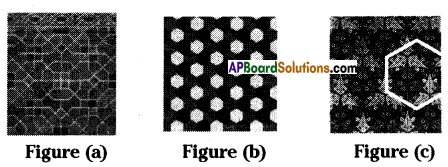AP State Syllabus AP Board 8th Class Maths Solutions Chapter 8 Exploring Geometrical Figures Ex 8.2 Textbook Questions and Answers.

## AP State Syllabus 8th Class Maths Solutions 8th Lesson Exploring Geometrical Figures Exercise 8.2Question 1.
Cut the bold type English alphabets (capital) and paste in your note book. Draw possible number of lines of symmetry for each of the letter.
(i) How many letters have no linear symmetry?
(ii) How many letters have one line of symmetry?
(iii) How many letters have two lines of symmetry?
(iv) How many letters have more than two lines of symmetry?
(v) Which of them have rotational symmetry?
(vi) Which of then have point symmetry?
Solution: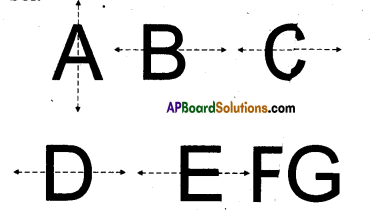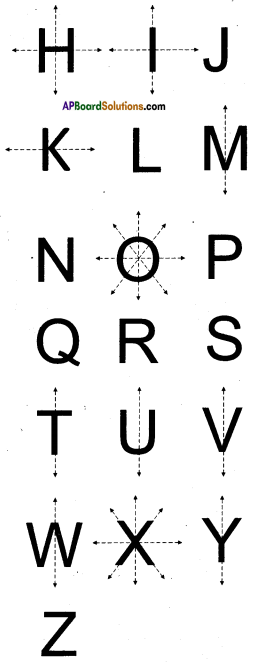i) letters don’t have line of symmetry
→ F, G, J, L, N, P, Q, R, S, Z.
ii) Liters which are having 1 line of symmetry
→ B, C, D, E, H, I, K, M, 0, T, U, V,
iii) Letirs which are having 2 lines of symmetry
→ H, I, O, X.
iv) Letters which are having more than two lines of symmetry O, X.
v) Letters which are having rotational symmetry
→ B, D, E, H, I, M, 0, S, T, W, X, Z.
vi) Letters which are having point symmetry
→ 0, X, M, W, H, 1, E, D.Question 2.
Draw lines of symmetry for the following figures. Identify which of them have point symmetry. Is there any implication between lines of symmetry and point symmetry?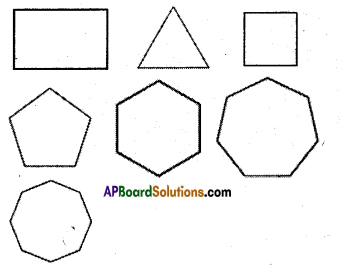Solution: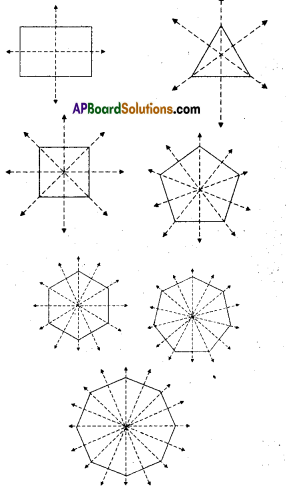The given figures has point of symmetry. Which are having line of symmetry are also having point of symmetry.Question 3.
Name some natural objects with faces which have atleast one line of symmetry.
Solution:
1) Moon (Full)
2) Face of a human being
3) Orange fruit
4) Sunflower
5) Butterfly

Question 4.
Draw three tessellations and name the basic shapes used on your tessellation.
Solution:
I may notice that the basic shapes used to draw tessellations are pentagon, rectangle, squares, equilateral triangles, hexagons etc. Most tessellations can’t be formed with these shapes.